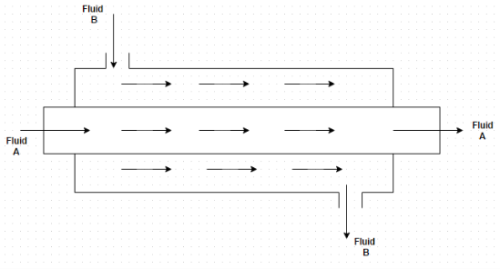# Test: Classification Of Heat Exchanger

## 10 Questions MCQ Test Heat Transfer for Engg. | Test: Classification Of Heat Exchanger

Description
This mock test of Test: Classification Of Heat Exchanger for Chemical Engineering helps you for every Chemical Engineering entrance exam. This contains 10 Multiple Choice Questions for Chemical Engineering Test: Classification Of Heat Exchanger (mcq) to study with solutions a complete question bank. The solved questions answers in this Test: Classification Of Heat Exchanger quiz give you a good mix of easy questions and tough questions. Chemical Engineering students definitely take this Test: Classification Of Heat Exchanger exercise for a better result in the exam. You can find other Test: Classification Of Heat Exchanger extra questions, long questions & short questions for Chemical Engineering on EduRev as well by searching above.
QUESTION: 1

### Some examples of heat exchanger are (i) Condensers and evaporators in refrigeration units (ii) Evaporator of an ice plant and milk chiller of a pasteurizing plant (iii) Automobile radiators and oil coolers of heat engines Identify the correct answer

Solution:

All are the examples of heat exchanger.

QUESTION: 2

### Heat exchangers are classified into how many categories?

Solution:

Nature of heat exchange process, relative direction of motion of fluid, mechanical design of heat exchange surface and physical state of heat exchanging fluids.

QUESTION: 3

### Based upon the nature of heat exchange process, the heat exchangers are classified into how many categories

Solution:

Direct contact, regenerators and recuperators.

QUESTION: 4

The energy transfer between the hot fluid and cold fluids is brought about by their complete physical mixing in

Solution:

In this type of heat exchanger, there is simultaneous transfer of heat and mass.

QUESTION: 5

Which type of flow arrangement is this?Solution:

In this type of arrangement, the fluids enter the unit from the same side, flow in the same direction and leave from the same side.

QUESTION: 6

Which of the following is not an example of recuperators type heat exchanger?

Solution:

Recuperators are not used in chemical factories.

QUESTION: 7

In how many categories heat exchangers are classified on the basis of direction of flow of fluids?

Solution:

Parallel, counter and cross flow.

QUESTION: 8

In how many categories heat exchangers are classified on the basis of mechanical design of heat exchanger surface?

Solution:

Concentric tubes, shell and tube and multiple shell.

QUESTION: 9

In how many categories heat exchangers are classified on the basis of physical state of heat exchanging fluids?

Solution:

Condenser and evaporator.

QUESTION: 10

Many types of heat exchangers have been developed to meet the widely varying applications. Based upon their
(i) Operating principle
(ii) Arrangement of flow path
(iii) Design
Identify the correct statements

Solution:

Heat exchanger is a process equipment designed for the effective transfer of heat energy between two fluids.